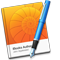# 鍵盤快速鍵

Option + 向左鍵

Option + 向右鍵

Command + 向左鍵

Command + 向右鍵

Option + 向上鍵

Option + 向下鍵

Command + E

Command + J

Command + 向上鍵

End

Command + 向下鍵

Command + A

Command + Shift + A

Shift + 向右鍵

Shift + 向左鍵

Shift + Option + 向右鍵

Shift + Option + 向左鍵

Command + Shift + 向右鍵

Command + Shift + 向左鍵

Shift + 向上鍵

Shift + 向下鍵

Shift + Option + 向上鍵

Shift + Option + 向下鍵

Command + Shift + 向上鍵

Command + Shift + 向下鍵，或 Shift + End

Command + T

Command + Shift + T

Command + Y

F1 到 F8（如果您已在「樣式」抽屜中指定它們）

Command + B

Command + I

Command + U

Command + Shift + 加號（+）

Command + Shift + 減號（–）

Command + Shift + Control + 加號（+）

Command + Shift + Control + 減號（–）

Command + Shift + 左括號（{）

Command + Shift + 垂直線（|）

Command + Shift + 右括號（}）

Command + Shift + Option + 垂直線（|）

Command + 左中括號（[）

Command + 右中括號（]）或 Tab

Command + X

Command + C

Command + Option + C

Command + Shift + Option + C

Command + V

Command + Option + V

Command + Shift + Option + V

Shift + 拖移或 Command + 拖移

Option + 空白鍵

Shift + Return

Return

Command + 按一下或 Shift + 按一下

Command + Option + C

Command + Option + V

Command + Shift + B

Command + Shift + Option + B

Command + Shift + F

Command + Shift + Option + F

Command + Option + G

Command + Shift + Option + G

Command + L

Command + Option + L

Command + D 或 Option + 拖移

Shift + 拖移

Option + 拖移選取控點

Shift + 拖移選取控點

Shift + Option + 拖移選取控點

Command + 拖移選取控點

Command + Shift + 拖移選取控點

Command + Option + 拖移選取控點

Command + Shift + Option + 拖移選取控點

Command + 拖移

Command + Shift + M

Return、Command + Return、Enter 或按兩下

Command + Return

Command + Shift + V

Command + Option + E

Command + Option + Control + T

Command + Option + Control + I

Option + 向上鍵

Option + 向下鍵

Option + 向右鍵

Option + 向左鍵

Command + A

Shift + 拖移表格

Option + 拖移所選輸入格到另一個輸入格

Return 或 Enter（在所選輸入格中）

Command + Return

Command + Return 兩次

Tab

Shift + Tab

Option + Tab（編輯文字時）

Control + Return

Option + Return

Return

Return 或 Enter

Esc（Escape）

Option + Return

Option + Tab

Command + Shift + D

Return

Shift + Return

Tab

Shift + Tab

Enter

End

Shift + End

Command + Shift + Option + P

Command + N

「樣板選擇器」打開新的書籍

Command + Shift + N

Command + O

Command + Option + P

Command + Shift + P

Command + S

Command + P

Command + Shift + 問號（?）

Command + F

Command + G

Command + Shift + G

Command + 分號（;）

Command + Shift + 冒號（:）

Command + W

Command + M

Command + Control + F

Command + 右角括號（>）

Command + 左角括號（<）

Command + 逗號（,）

Command + Option + I

Command + R

Command + Shift + L

Command + Option + R

Command + Shift + I

Command + Shift + C

Command + Option + T

Command + Shift + R

Command + Shift + E

Command + H

Command + Option + H

Command + Z

Command + Shift + Z

Command + Q